1. /
2. CBSE
3. /
4. Class 05
5. /
6. Mathematics
7. /
8. NCERT Solutions for Class...

# NCERT Solutions for Class 6 Maths Exercise 10.3

NCERT solutions for Maths Mensuration## NCERT Solutions for Class 6 Maths Mensuration

###### Question 1.Find the areas of the rectangles whose sides are:

(a) 3 cm and 4 cm

(b) 12 m and 21 m

(c) 2 km and 3 km

(d) 2 m and 70 cm

(a) Area of rectangle = length x breadth

= 3 cm x 4 cm = 12 cm2

(b) Area of rectangle = length x breadth

= 12 m x 21 m = 252 m2

(c) Area of rectangle = length x breadth

= 2 km x 3 km = 6 km2

(d) Area of rectangle = length x breadth

= 2 m x 70 cm = 2 m x 0.7 m = 1.4 m2

###### Question 2.Find the areas of the squares whose sides are:

(a) 10 cm

(b) 14 cm

(c) 5 cm

(a) Area of square = side x side = 10 cm x 10 cm = 100 cm2

(b) Area of square = side x side = 14 cm x 14 cm = 196 cm2

(c) Area of square = side x side = 5 m x 5 m = 25 m2

###### Question 3.The length and the breadth of three rectangles are as given below:

(a) 9 m and 6 m

(b) 17 m and 3 m

(c) 4 m and 14 m

Which one has the largest area and which one has the smallest?

(a) Area of rectangle = length x breadth = 9 m x 6 m = 54 m2

(b) Area of rectangle = length x breadth= 3 m x 17 m = 51 m2

(c) Area of rectangle = length x breadth= 4 m x 14 m = 56 m2

Thus, the rectangle (c) has largest area, i.e. 56 m2 and rectangle (b) has smallest area, i.e., 56 m2.

NCERT Solutions for Class 6 Maths Exercise 10.3

###### Question 4.The area of a rectangle garden 50 m long is 300 m2, find the width of the garden.

Length of rectangle = 50 m and Area of rectangle = 300 m2

Since, Area of rectangle = length x breadth

Therefore, Breadth = {tex}\frac{{{\text{Area of rectangle}}}}{{{\text{Length}}}}{/tex} = = 6 m

Thus, the breadth of the garden is 6 m.

###### Question 5.What is the cost of tilling a rectangular plot of land 500 m long and 200 m wide at the rate of Rs. 8 per hundred sq. m?

Length of land = 500 m and Breadth of land = 200 m

Area of land = length x breadth = 500 m x 200 m = 1,00,000 m2

{tex}\because {/tex} Cost of tilling 100 sq. m of land = Rs. 8

{tex}\therefore {/tex} Cost of tilling 1,00,000 sq. m of land = = Rs. 8000

NCERT Solutions for Class 6 Maths Exercise 10.3

###### Question 6.A table-top measures 2 m by 1 m 50 cm. What is its area in square meters?

Answer: Length of table = 2 m and breadth of table = 1 m 50 cm = 1.50 m

Area of table = length x breadth

= 2 m x 1.50 m = 3 m2

###### Question 7.A room us 4 m long and 3 m 50 cm wide. How many square meters of carpet is needed to cover the floor of the room?

Answer: Length of room = 4 m and breadth of room = 3 m 50 cm = 3.50 m

Area of carpet = length x breadth

= 4 x 3.50 = 14m2

NCERT Solutions for Class 6 Maths Exercise 10.3

###### Question 8.A floor is 5 m long and 4 m wide. A square carpet of sides 3 m is laid on the floor. Find the area of the floor that is not carpeted.

Answer: Length of floor = 5 m and breadth of floor = 4 m

Area of floor = length x breadth

= 5 m x 4 m = 20 m2

Now, Side of square carpet = 3 m

Area of square carpet = side x side = 3 x 3 = 9 m2

Area of floor that is not carpeted = 20 m2 – 9 m2 = 11 m2

###### Question 9.Five square flower beds each of sides 1 m are dug on a piece of land 5 m long and 4 m wide. What is the area of the remaining part of the land?

Answer: Side of square bed = 1 m

Area of square bed = side x side = 1 m x 1 m = 1 m2

{tex}\therefore {/tex} Area of 5 square beds = 1 x 5 = 5 m2

Now, Length of land = 5 m and breadth of land = 4 m

{tex}\therefore {/tex} Area of land = length x breadth = 5 m x 4 m = 20 m2

Area of remaining part = Area of land – Area of 5 flower beds

= 20 m2 – 5 m2 = 15 m2

NCERT Solutions for Class 6 Maths Exercise 10.3

###### Question 10.By splitting the following figures into rectangles, find their areas. (The measures are given in centimeters)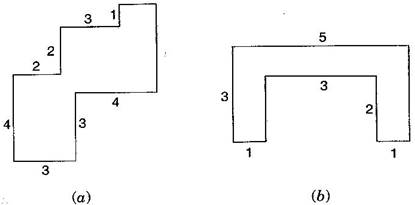(a) Area of HKLM = 3 x 3 = 9 cm2

Area of IJGH = 1 x 2 = 2 cm2

Area of FEDG = 3 x 3 = 9 cm2

Area of ABCD = 2 x 4 = 8 cm2

Total area of the figure = 9 + 2 + 9 + 8 = 28 cm2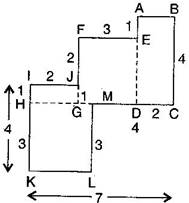(b) Area of ABCD = 3 x 1 = 3 cm2

Area of BDEF = 3 x 1 = 3 cm2

Area of FGHI = 3 x 1 = 3 cm2

Total area of the figure = 3 + 3 + 3 = 9 cm2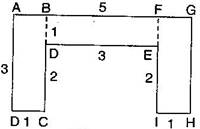###### Question 11.Split the following shapes into rectangles and find their areas. (The measures are given in centimeters)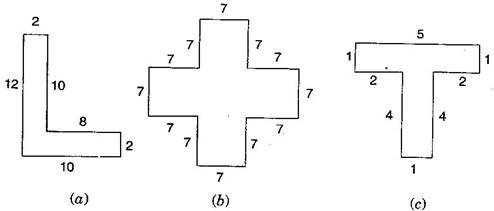(a) Area of rectangle ABCD = 2 x 10 = 20 cm2

Area of rectangle DEFG = 10 x 2 = 20 cm2

Total area of the figure = 20 + 20 = 40 cm2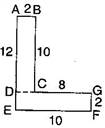(b) There are 5 squares each of side 7 cm.

Area of one square = 7 x 7 = 49 cm2

Area of 5 squares = 49 x 5 = 245 cm2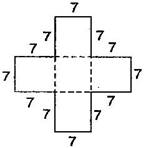(c) Area of rectangle ABCD = 5 x 1 = 5 cm2

Area of rectangle EFGH = 4 x 1 = 4 cm2

Total area of the figure = 5 + 4 cm2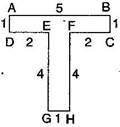NCERT Solutions for Class 6 Maths Exercise 10.3

###### Question 12.How many tiles whose length and breadth are 12 cm and 5 cm respectively will be needed to fit in a rectangular region whose length and breadth are respectively:

(a)100 cm and 144 cm

(b)70 cm and 36 cm

(a) Area of region = 100 cm x 144 cm = 14400 cm2

Area of one tile = 5 cm x 12 cm = 60 cm2

Number of tiles = {tex}\frac{{{\text{Area of region}}}}{{{\text{Area of one tile}}}}{/tex}

= 240

Thus, 240 tiles are required.

(b) Area of region = 70 cm x 36 cm = 2520 cm2

Area of one tile = 5 cm x 12 cm = 60 cm2

Number of tiles = {tex}\frac{{{\text{Area of region}}}}{{{\text{Area of one tile}}}}{/tex}

= 42

Thus, 42 tiles are required.

## NCERT Solutions for Class 6 Maths Exercise 10.3

NCERT Solutions Class 6 Maths PDF (Download) Free from myCBSEguide app and myCBSEguide website. Ncert solution class 6 Maths includes text book solutions from Class 6 Maths Book . NCERT Solutions for CBSE Class 6 Maths have total 14 chapters. 6 Maths NCERT Solutions in PDF for free Download on our website. Ncert Maths class 6 solutions PDF and Maths ncert class 6 PDF solutions with latest modifications and as per the latest CBSE syllabus are only available in myCBSEguide.

## CBSE app for Students

To download NCERT Solutions for Class 6 Maths, Social Science Computer Science, Home Science, Hindi English, Maths Science do check myCBSEguide app or website. myCBSEguide provides sample papers with solution, test papers for chapter-wise practice, NCERT solutions, NCERT Exemplar solutions, quick revision notes for ready reference, CBSE guess papers and CBSE important question papers. Sample Paper all are made available through the best app for CBSE students and myCBSEguide website.### Test Generator

Create question paper PDF and online tests with your own name & logo in minutes.### myCBSEguide

Question Bank, Mock Tests, Exam Papers, NCERT Solutions, Sample Papers, Notes

### 20 thoughts on “NCERT Solutions for Class 6 Maths Exercise 10.3”

1. Very good
Thanks a lot

2. In every site every photo had blure in this site every thing is clear

3. Very good

4. good

5. Thank you for providing such a good solutions all doubts are cleared thanks a lot for solving the students doubts thank you .

6. thank you for giving this solution thank you very much

7. Thanks alot for clearing our doubts.

8. Thanks alot for clearing our doubts.And this one is very useful us. I may thought that this is the better way to help as.

9. This one is very useful us. I may thought that this is the better way to help as.

10. It just a nice site it clear all my doubt

11. I like this ??

12. It’s very useful
Thank you

13. Thanks & it’s good

14. thanks yaar mujhe laga answer nahi milega

15. Thanks very much

16. It is very good and useful for kids

17. the video is is very knowledgefull